﻿ 基于动网格技术研究海流对水下机器人运动特性的影响
 舰船科学技术2017, Vol. 39Issue (12): 20-25PDF

Study the effect of ocean current on the motion characteristics of underwater vehicle based on dynamic grid technology
WU Jia-ming, ZHONG Le, ZHANG En-wei, WANG Sheng-yong
School of Civil Engineering and Transportation, South China University of Technology, Guangzhou 510640, China
Abstract: Based on the 6DOF solver provided by Fluent, using the dynamic grid technique to solved the N-S equation by computational fluid dynamics method in the basin where the underwater robot system is located, research the motion characteristics of underwater robot under the influence of current. The numerical results show that, using dynamic grid technique to the 6DOF motion simulation can obtain the influence of current on the motion characteristics of underwater robot. With the increase of current velocity, the instability of underwater robot movement increases gradually, and there will be obvious yaw in the later period of motion, the distance of yaw occurs with the increase of ocean velocity. With the increase of thrust, the curvature of the displacement trajectory curve decreases gradually, transition from curve to straight line gradually.
Key words: currents     underwater robot     6DOF     dynamic mesh     movement characteristics
0 引　言

1 数学模型及计算方法 1.1 控制方程与湍流模型

 $\frac{{\partial {u_x}}}{{\partial x}} + \frac{{\partial {u_y}}}{{\partial y}} + \frac{{\partial {u_z}}}{{\partial z}} = 0\text{，}$ (1)

 \begin{aligned}\rho \left( {\frac{{\partial {{\rm{u}}_x}}}{{\partial t}} + {u_x}\frac{{\partial {{\rm{u}}_x}}}{{\partial x}} + {u_y}\frac{{\partial {{\rm{u}}_x}}}{{\partial y}} + {u_z}\frac{{\partial {{\rm{u}}_x}}}{{\partial z}}} \right) =\\- \frac{{\partial p}}{{\partial x}} + \nu \rho \left( {\frac{{{\partial ^2}{{\rm{u}}_x}}}{{\partial {x^2}}} + \frac{{{\partial ^2}{{\rm{u}}_x}}}{{\partial {y^2}}} + \frac{{{\partial ^2}{{\rm{u}}_x}}}{{\partial {z^2}}}} \right)\text{，}\end{aligned} (2)
 \begin{aligned}\rho \left( {\frac{{\partial {{\rm{u}}_y}}}{{\partial t}} + {u_x}\frac{{\partial {{\rm{u}}_y}}}{{\partial x}} + {u_y}\frac{{\partial {{\rm{u}}_y}}}{{\partial y}} + {u_z}\frac{{\partial {{\rm{u}}_y}}}{{\partial z}}} \right) =\\- \frac{{\partial p}}{{\partial y}} + \nu \rho \left( {\frac{{{\partial ^2}{{\rm{u}}_y}}}{{\partial {x^2}}} + \frac{{{\partial ^2}{{\rm{u}}_y}}}{{\partial {y^2}}} + \frac{{{\partial ^2}{{\rm{u}}_y}}}{{\partial {z^2}}}} \right)\text{，}\end{aligned} (3)
 \begin{aligned}\rho \left( {\frac{{\partial {{\rm{u}}_{\rm{z}}}}}{{\partial t}} + {u_x}\frac{{\partial {{\rm{u}}_z}}}{{\partial x}} + {u_y}\frac{{\partial {{\rm{u}}_z}}}{{\partial y}} + {u_z}\frac{{\partial {{\rm{u}}_z}}}{{\partial z}}} \right) =\\- \frac{{\partial p}}{{\partial z}} + \nu \rho \left( {\frac{{{\partial ^2}{{\rm{u}}_z}}}{{\partial {x^2}}} + \frac{{{\partial ^2}{{\rm{u}}_z}}}{{\partial {y^2}}} + \frac{{{\partial ^2}{{\rm{u}}_z}}}{{\partial {z^2}}}} \right)\text{。}\end{aligned} (4)

 $\begin{split}& \frac{{\partial \rho k}}{{\partial t}} + \frac{\partial }{{\partial {x_j}}}(\rho {u_j}k) = - \frac{2}{3}\rho k\frac{{\partial {u_k}}}{{\partial {x_j}}} +\\ &\frac{\partial }{{\partial {x_j}}}(\frac{{{\mu _{eff}}}}{{{\sigma _k}}}\frac{{\partial k}}{{\partial {x_j}}}) + {G_k} - \rho \varepsilon \text{，}\end{split}$ (5)
 $\begin{array}{l}\displaystyle\frac{{\partial \rho \varepsilon }}{{\partial t}} + \displaystyle\frac{\partial }{{\partial {x_j}}}(\rho {u_j}\varepsilon ) = - [(\displaystyle\frac{2}{3}{C_{\varepsilon 1}} - {C_{\varepsilon 3}} + \displaystyle\frac{2}{3}{C_\mu }{C_\eta }\displaystyle\frac{{k\partial {u_k}}}{{\varepsilon \partial {x_k}}})\rho \varepsilon \displaystyle\frac{{\partial {u_k}}}{{\partial {x_k}}}\\ \quad\quad+ \displaystyle\frac{\partial }{{\partial {x_j}}}(\displaystyle\frac{{{\mu _{eff}}}}{{{\sigma _\varepsilon }}}\displaystyle\frac{{\partial \varepsilon }}{{\partial {x_j}}})] + \rho \displaystyle\frac{\varepsilon }{k}[({C_{\varepsilon 1}} - {C_\eta }){C_k} - {C_{\varepsilon 2}}\rho \varepsilon ]\text{，}\ \; \, \end{array}$ (6)

1.2 水下机器人系统几何模型构建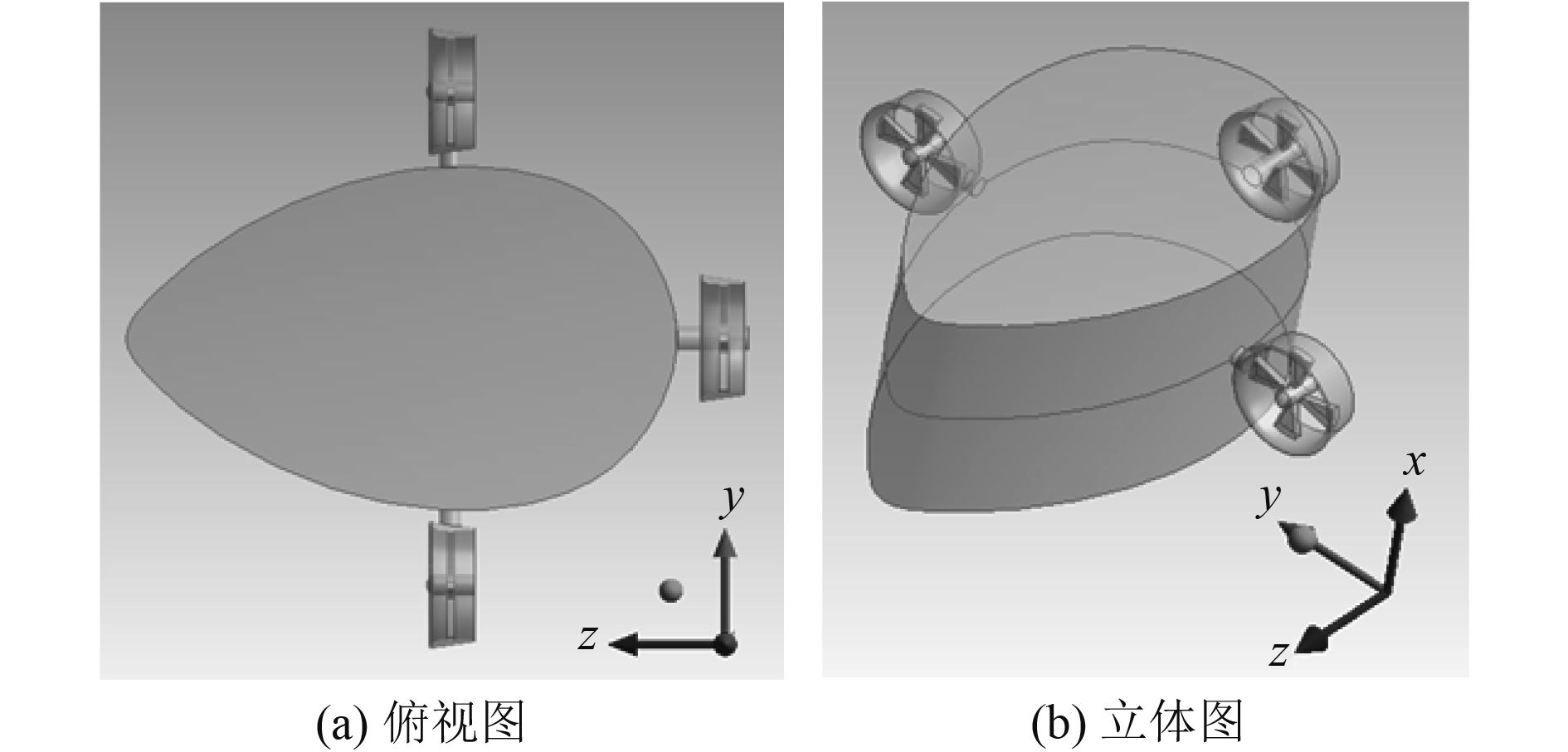图 1 导管螺旋桨几何模型 Fig. 1 Geometric model of catheter propeller
1.3 计算域网格与边界条件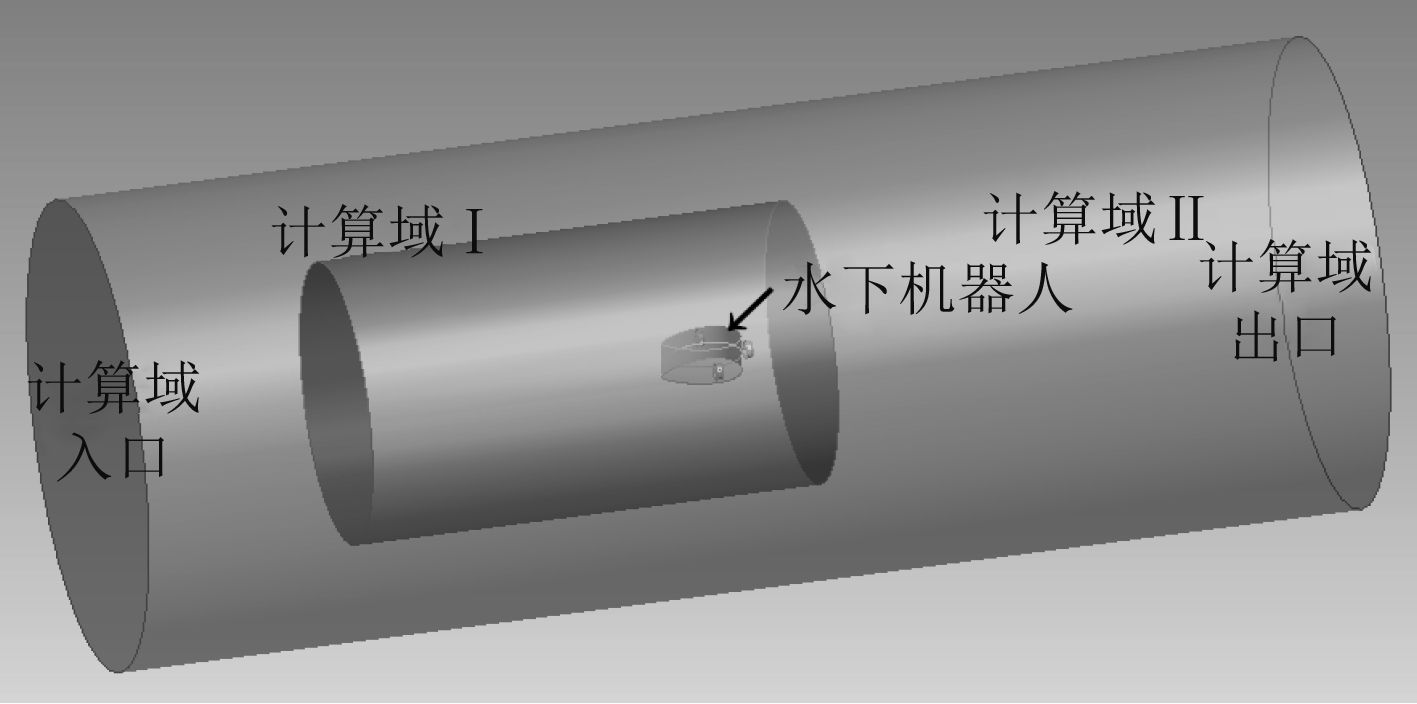图 2 水下机器人系统计算域 Fig. 2 Computing domain of underwater robot system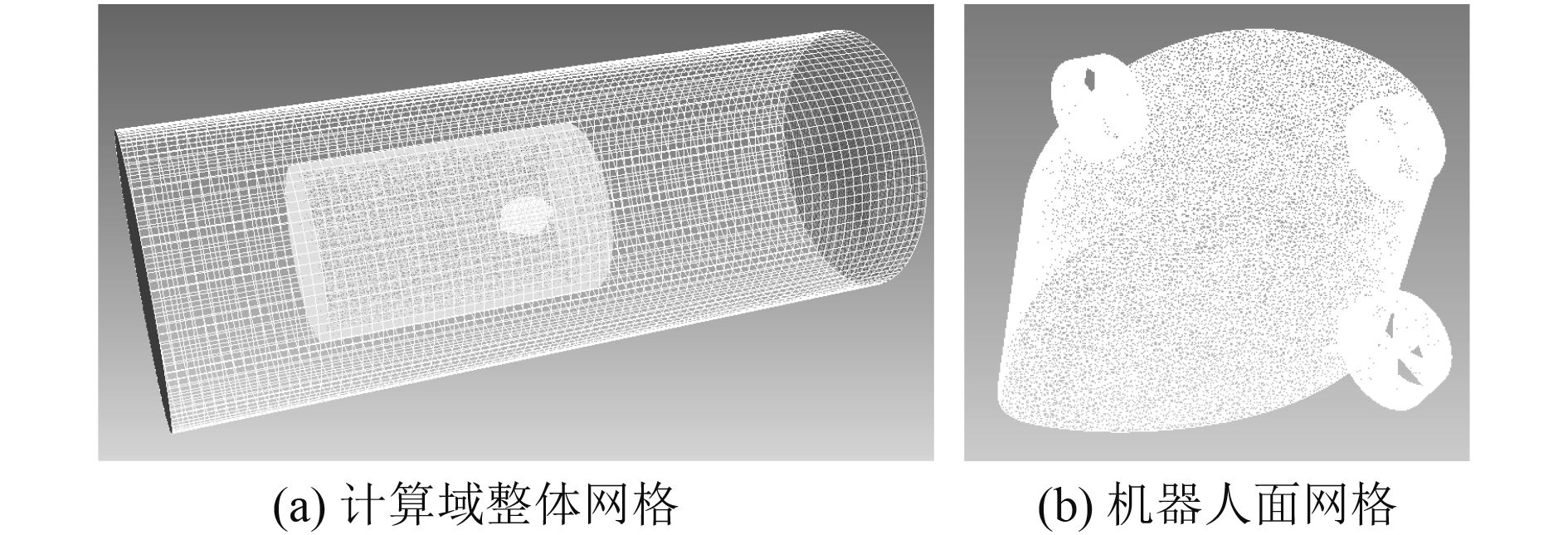图 3 水下机器人系统计算域网格 Fig. 3 Computing domain grid of underwater robot system表 1 水下机器人系统计算域网格信息 Tab.1 Computing domain grid information of underwater robot system表 2 水下机器人系统边界条件 Tab.2 Boundary conditions of underwater robot system

1.4 网格更新方式

1.5 6DOF计算方法

Fluent中的6DOF计算模型用于计算刚体在流体作用下的运动参数（速度、角速度等）、运动轨迹（重心位置）。在该模型中，物体或边界的运动是由作用在其上的力、力矩以及其自身所受的重力、初始推力等外力的共同作用决定。所以物体的运动与流场的计算相互耦合，这种运动我们称之为耦合运动。

2 数值模拟结果

2.1 逆向来流、无转矩恒定推力作用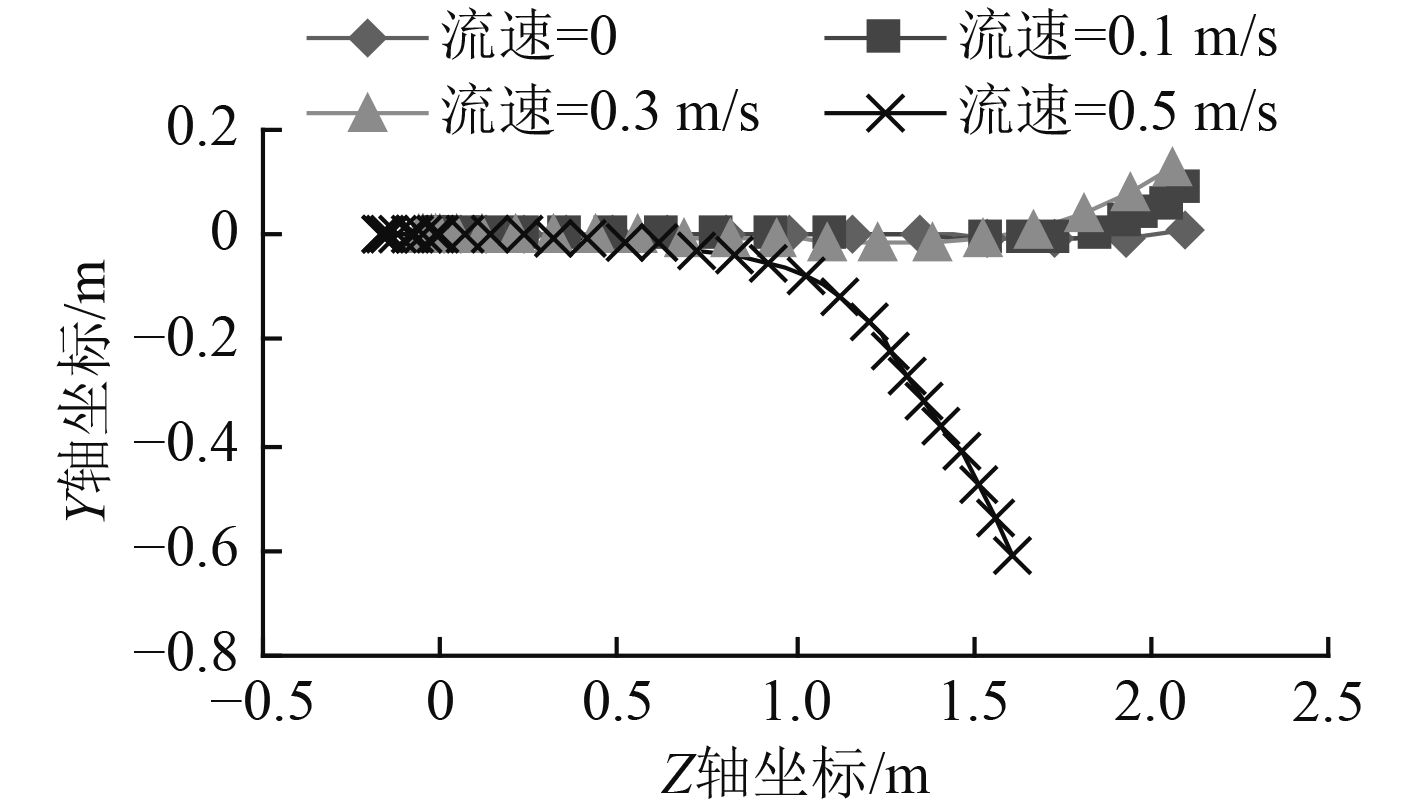图 4 逆向来流轨迹曲线 Fig. 4 Trajectory curve under reverse flow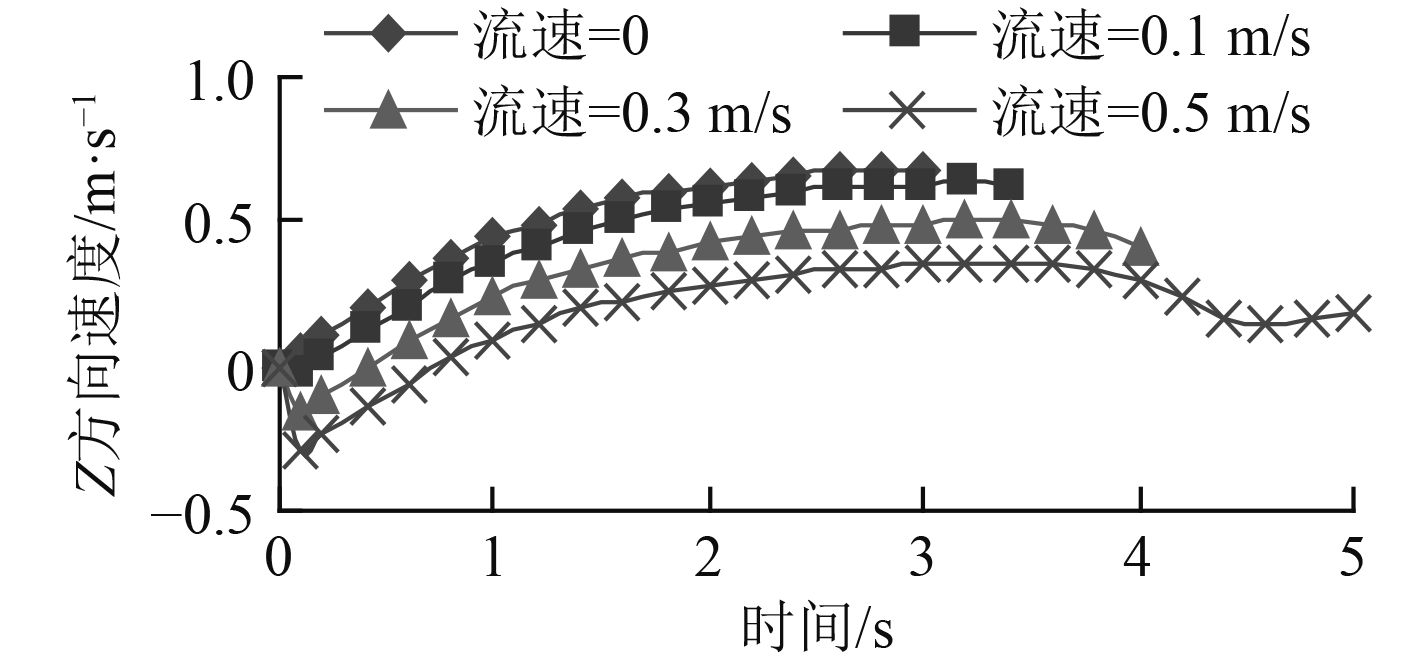图 5 逆向来流Z方向速度曲线 Fig. 5 Z direction velocity curve under reverse flow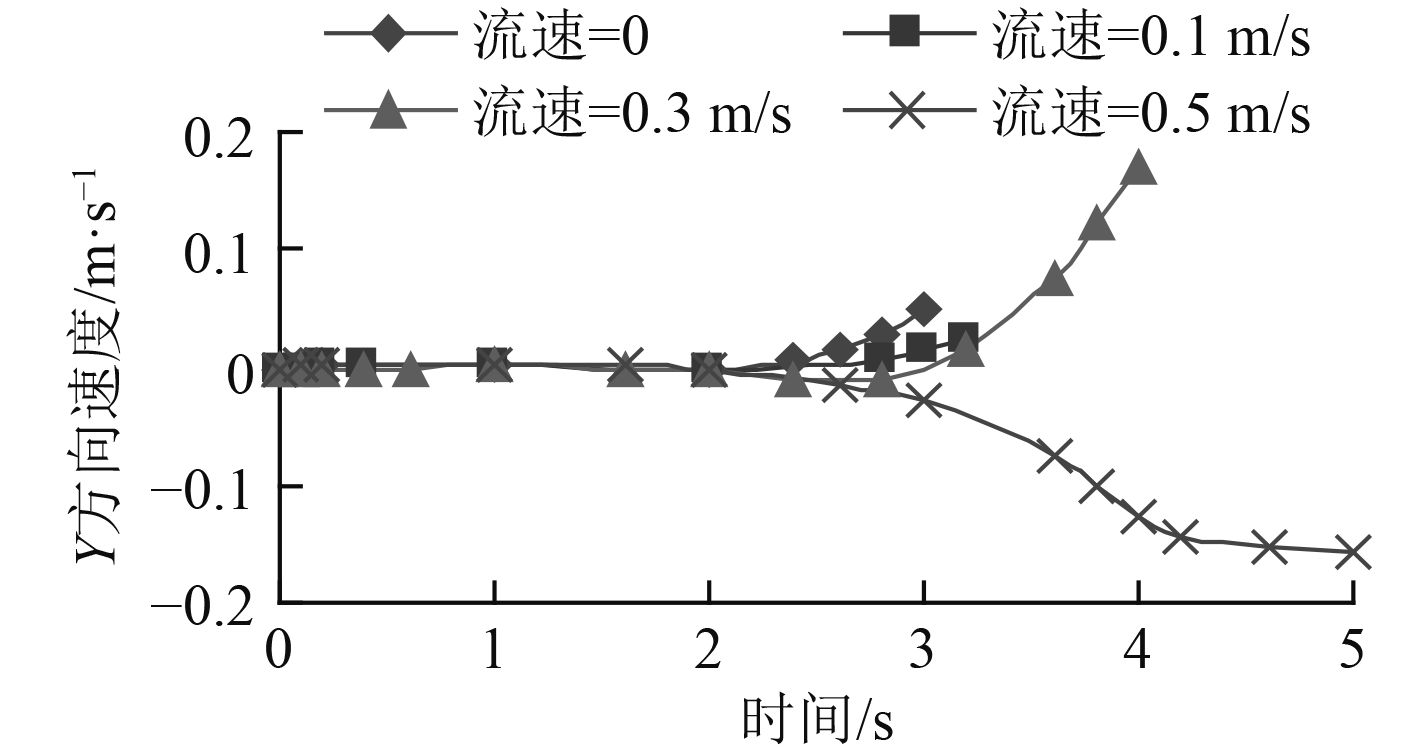图 6 逆向来流Y方向速度曲线 Fig. 6 Y direction velocity curve under reverse flow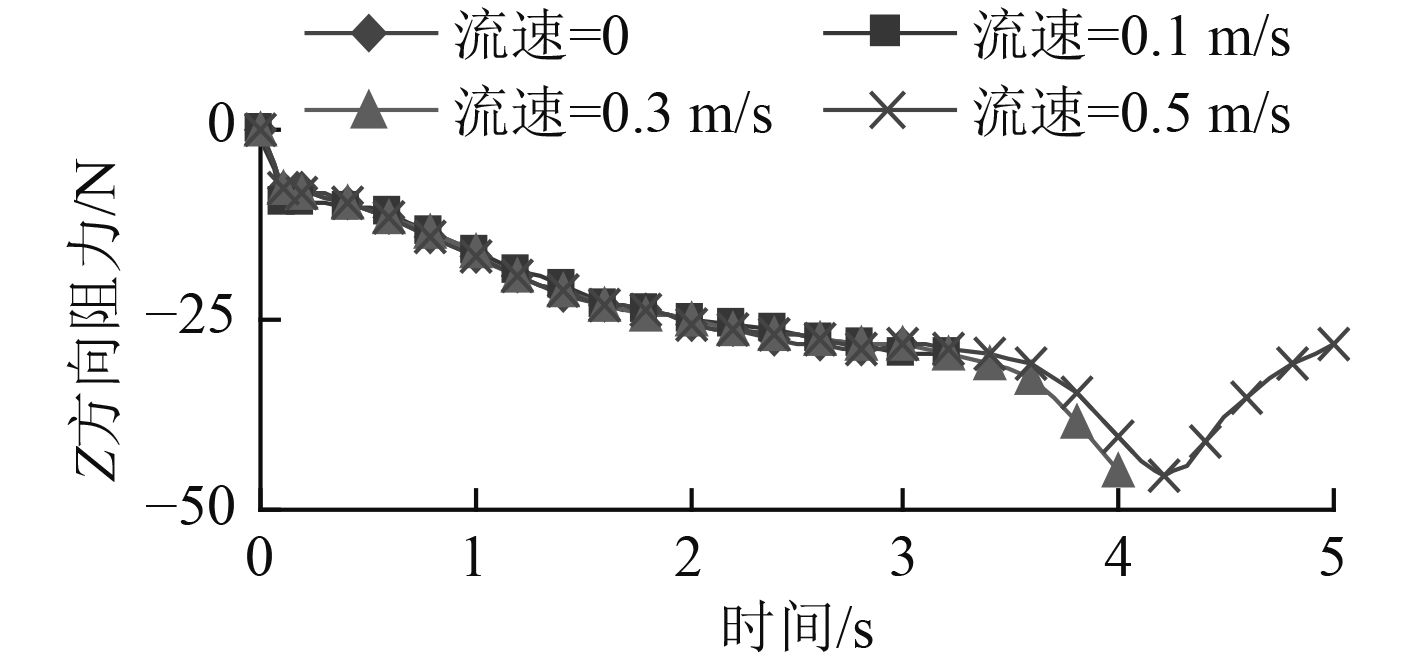图 7 逆向来流Z方向阻力曲线 Fig. 7 Z direction resistance curve under reverse flow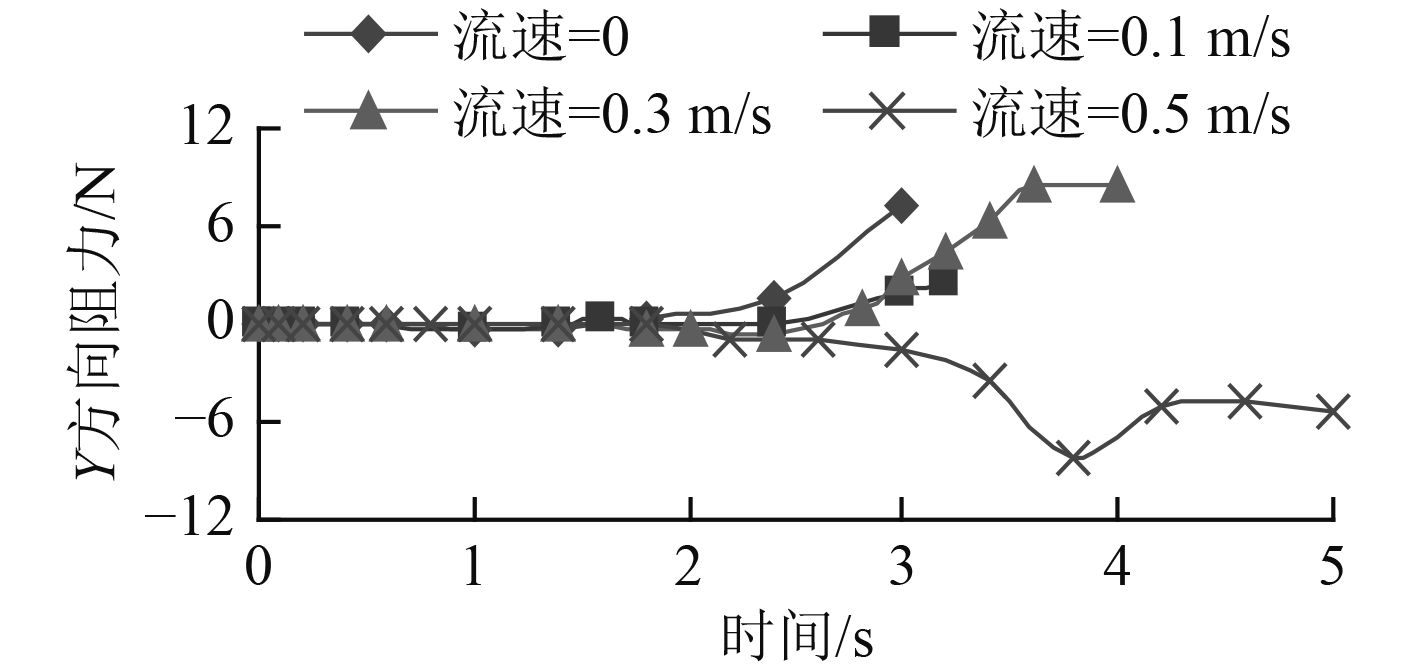图 8 逆向来流Y方向阻力曲线 Fig. 8 Y direction resistance curve under reverse flow

2.2 顺向来流、无转矩恒定推力作用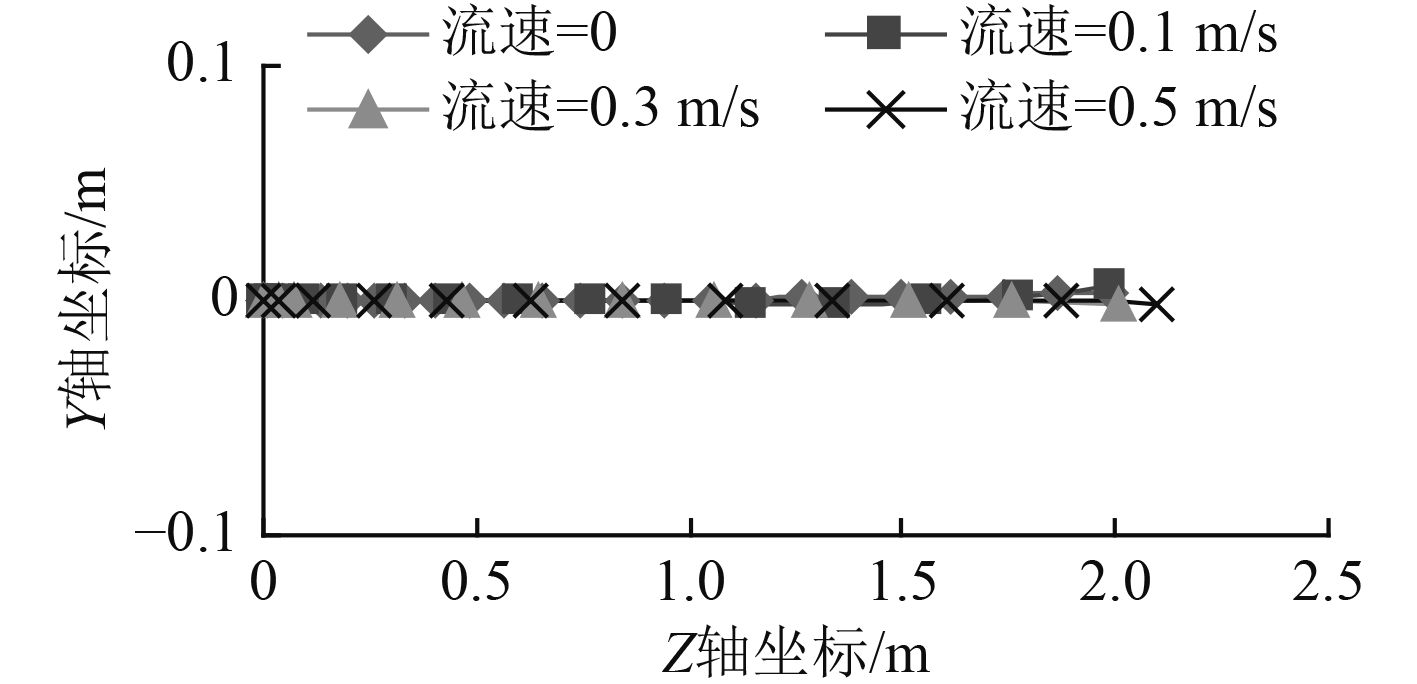图 9 顺向来流轨迹曲线 Fig. 9 Trajectory curve under forward flow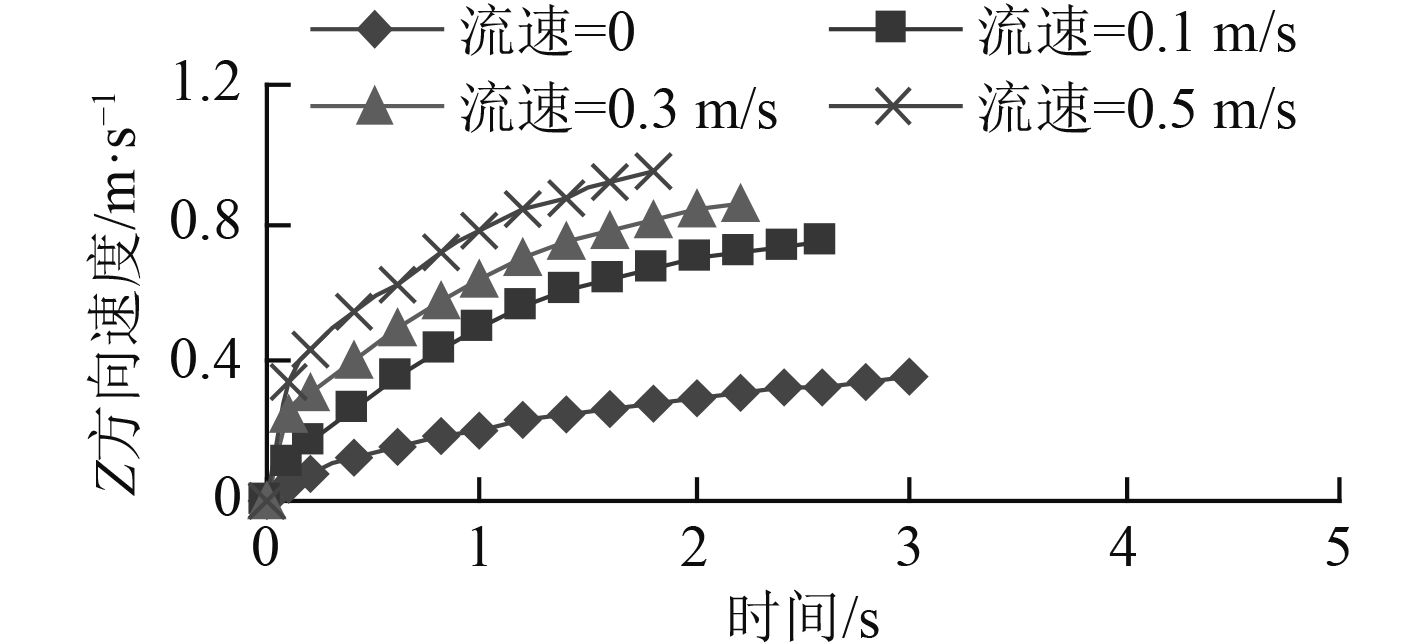图 10 顺向来流Z方向速度曲线 Fig. 10 Z direction velocity curve under forward flow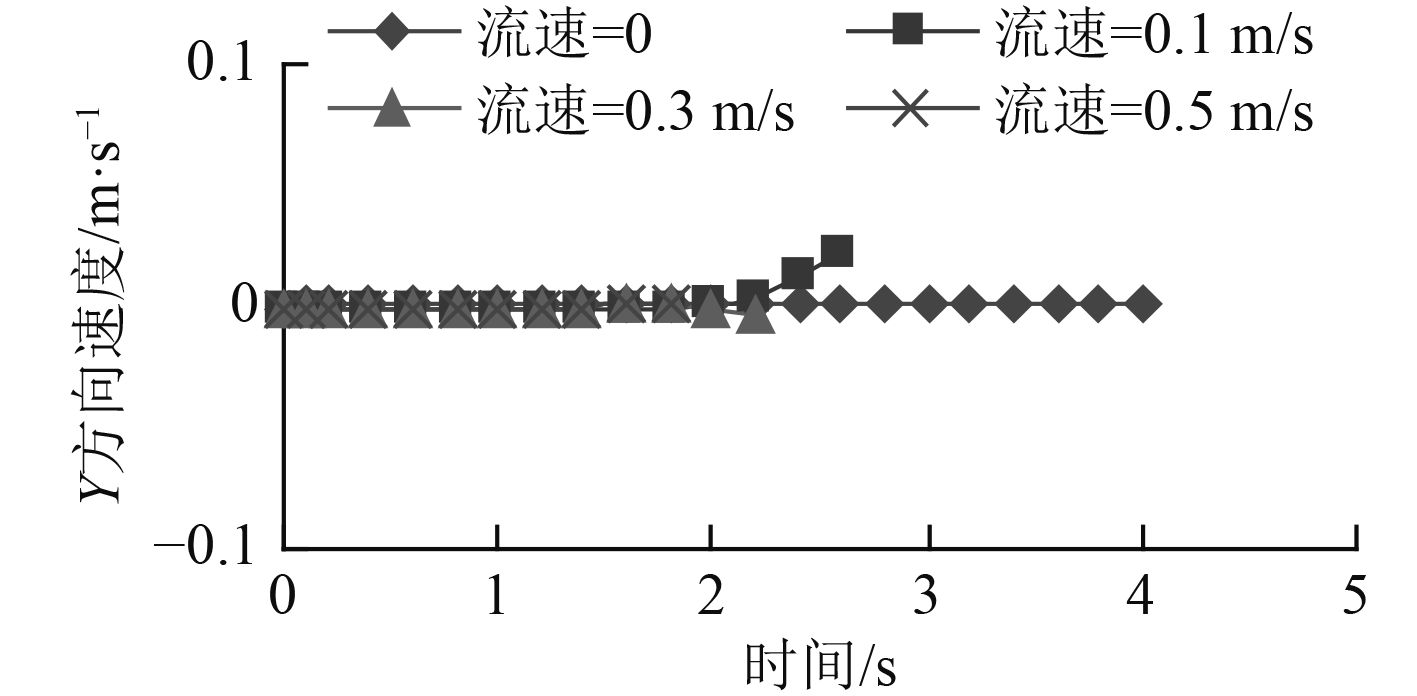图 11 顺向来流Y方向速度曲线 Fig. 11 Y direction velocity curve under forward flow

2.3 逆向来流、恒定转矩变推力作用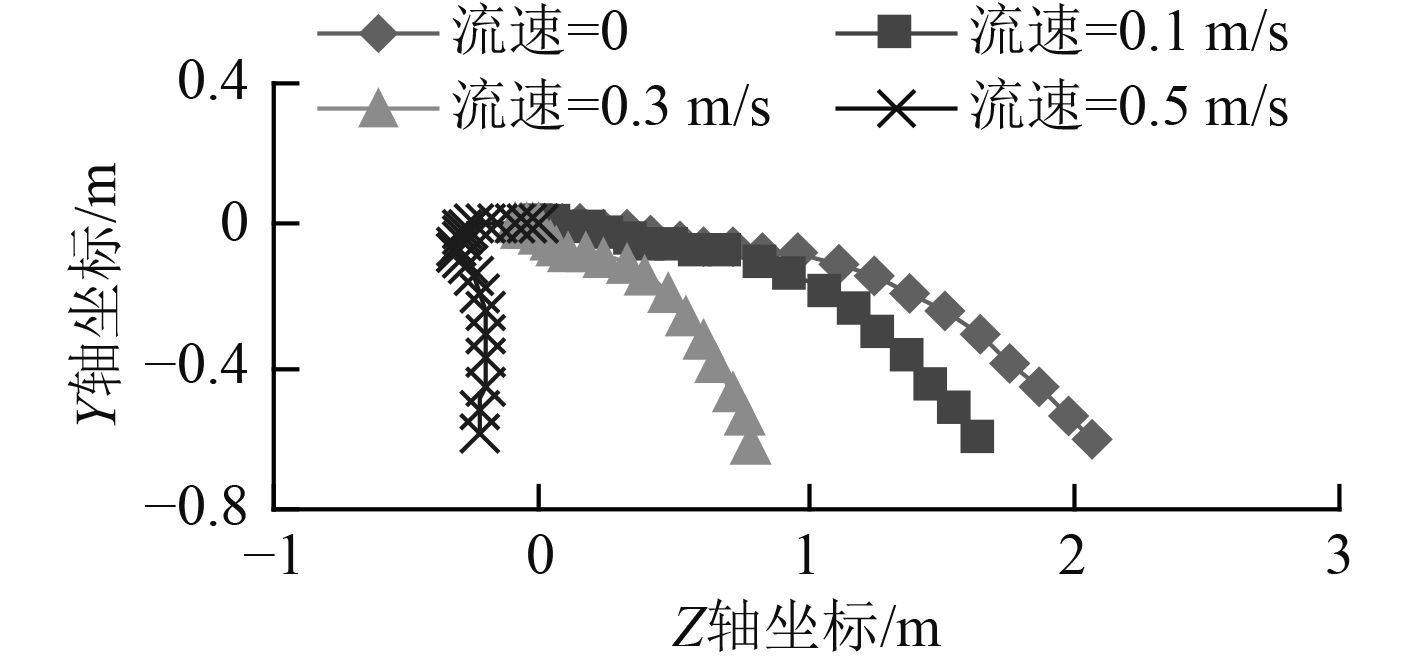图 12 推力为20 N轨迹曲线 Fig. 12 Trajectory curve under thrust is 20 N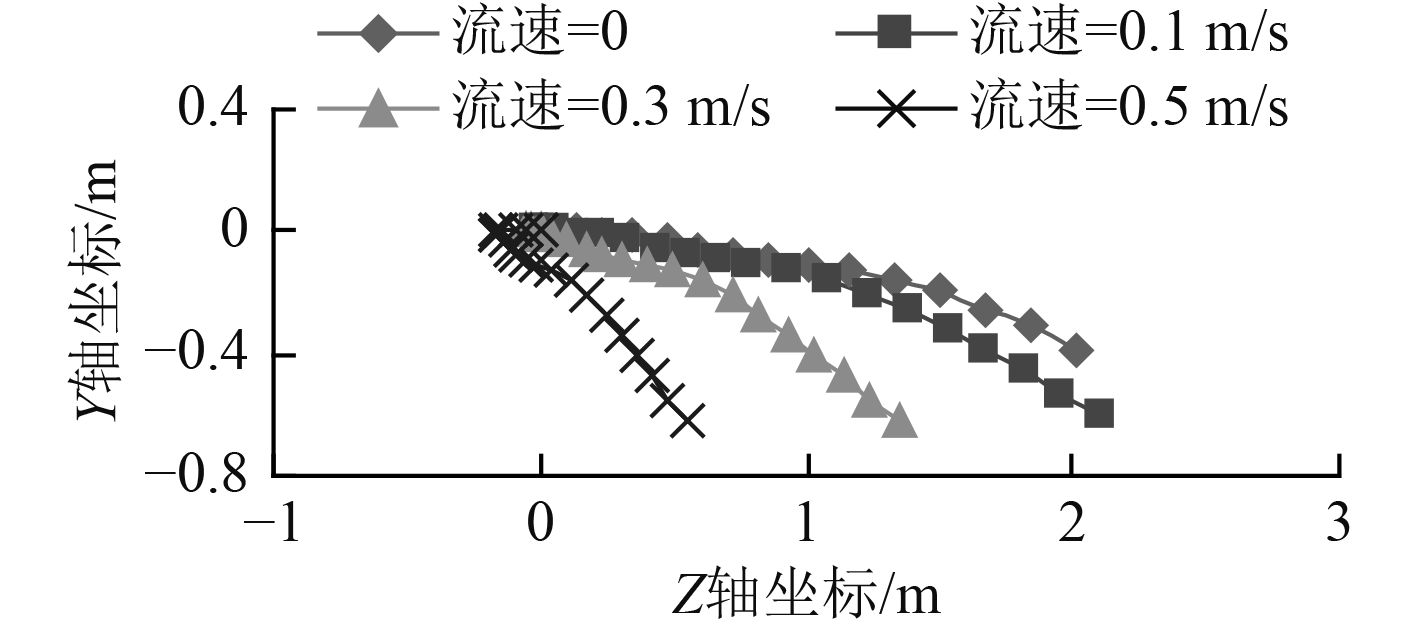图 13 推力为30 N轨迹曲线 Fig. 13 Trajectory curve under thrust is 30 N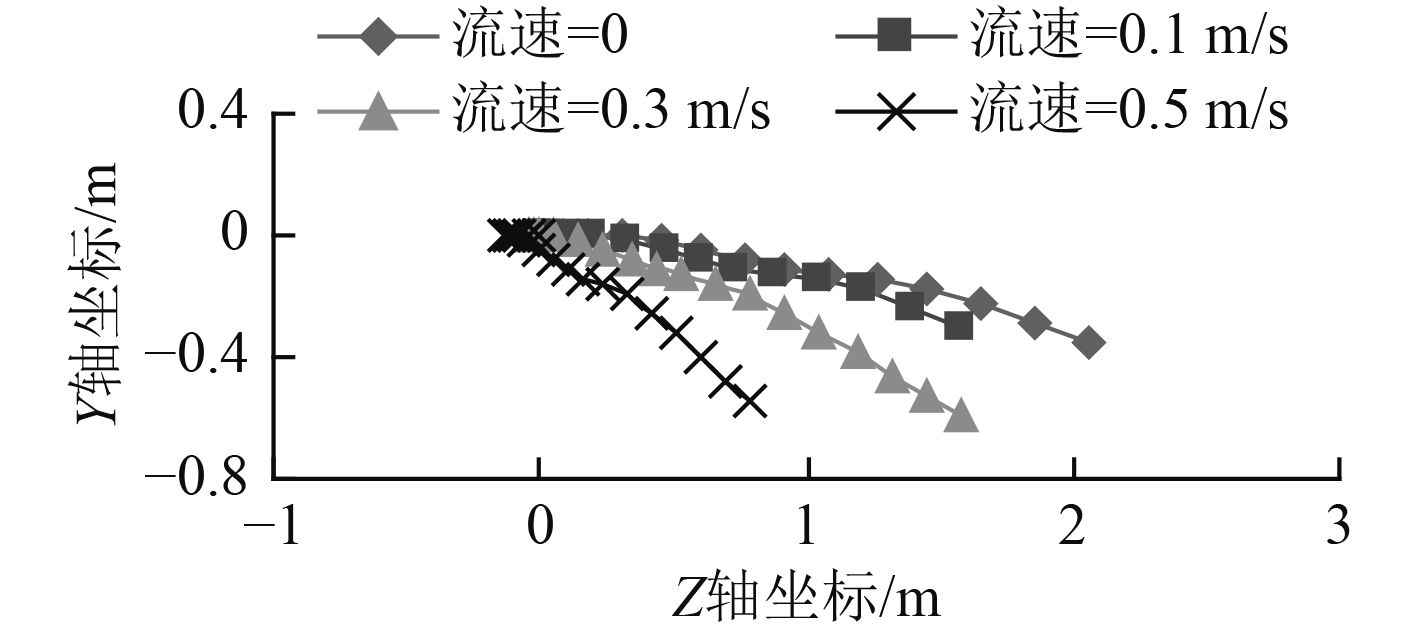图 14 推力为40 N轨迹曲线 Fig. 14 Trajectory curve under thrust is 40 N

3 结　语

1）基于动网格技术，采用弹簧光顺与局部网格重构的组合式网格更新方式，以六自由度（6DOF）运动方式来模拟水下机器人不同的受力情况、不同的海流速度下的运动行之有效，可以获得水下机器人的运动规律。

2）不同海流方向、不同海流速度大小、不同受力情况下，水下机器人的运动规律表现出明显的不同：随着海流速度的增加，水下机器人运动的不稳定性逐渐增加，在运动后期会出现明显的偏航，且发生偏航的距离点随着海流速度的增加而提前；随着推力的增加，位移轨迹曲线、速度曲线的曲度逐渐减小，逐渐由曲线过渡为直线；而且，随着运动时间的推进，水下机器人的运动趋向于平稳；顺向海流影响时，水下机器人运动稳定性明显高于逆向海流，相同推力下的运动速度、加速度都较逆向海流时大。

  李慧, 赵琳, 毛英. 海况干扰下潜艇六自由度运动分析[J]. 哈尔滨工程大学学报, 2015, 31 (4): 1–7.  吴家鸣, 邓威, 赖华威. 回转状态下导管螺旋桨水动力特性的数值模拟[J]. 华南理工大学学报: 自然科学版, 2010, 38 (7): 90–96. WU Jia-ming, DENG Wei, LAI Hua-wei. Numerical simulation of hydrodynamic characteristics of ducted propeller in turning motion[J]. Journal of South China University of Technology (Natural Science Edition), 2010, 38 (7): 90–96.  吴家鸣, 叶志坚, 金晓东, 等. 水下潜器导管螺旋桨在转艏摆动中的推力特性分析[J]. 华南理工大学学报: 自然科学版, 2015, 43 (12): 141–148. WU Jia-ming, YE Zhi-jian, JIN Xiao-dong, et al. Analysis of thrust characteristics of ducted propellers in underwater vehicle with yawing motion[J]. Journal of South China University of Technology (Natural Science Edition), 2015, 43 (12): 141–148.  张宏伟, 王树新, 侯巍, 等. 螺旋桨三维建模方法研究[J]. 机床与液压, 2006 (5): 60–62.  李迎华, 吴宝山, 张华. CFD动态网格技术在水下航行体非定常操纵运动预报中的应用研究[J]. 船舶力学, 2010, 14 (10): 1100–1108. LI Ying-Hua, WU Bao-Shan, ZHANG Hua. Research on application of prediction for unsteady maneuvering motion of underwater vehicle by dynamic mesh technique in CFD[J]. Journal of Ship Mechanics, 2010, 14 (10): 1100–1108. DOI: 10.3969/j.issn.1007-7294.2010.10.004  王福军. 计算流体动力学分析—CFD软件原理与应用[M]. 北京: 清华大学出版社, 2004: 1–4.  韩占忠, 王敏, 兰小平. FLUENT流体工程仿真计算实例与应用[M]. 北京: 北京理工大学出版社, 2004: 15.  吴家鸣, 钟乐, 张恩伟, 等. 水下机器人进行逆向运动时导管螺旋桨推力特性的模拟[J]. 船舶工程, 2017, 39 (S1): 225–229. WU Jia-ming, ZHONG Le, ZHANG En-we, et al. Study on hydrodynamics of underwater robot in reverse propeller and negative speed[J]. Ship Engineering, 2017, 39 (S1): 225–229.  ANTONELLI G. On the use of adaptive/integral actions for six-degrees-of- freedom control of autonomous underwater vehicles[J]. IEEE Journal of Oceanic Engineering, 2007, 32 (2): 300–312. DOI: 10.1109/JOE.2007.893685  张来平, 邓小光, 张涵信. 动网格生成技术及非定常计算方法进展综述[J]. 力学进展, 2010, 40 (4): 424–447. DOI: 10.6052/1000-0992-2010-4-J2009-123  隋洪涛, 李鹏飞, 马世虎. 精通CFD动网格工程仿真与案例实战 [M]. 北京: 人民邮电出版社, 2013:5.  He ZHANG, Yu-ru XU, Hao-peng CAI. Using CFD Software to Calculate Hydrodynamic Coefficients[J]. Journal of Marine Science and Application, 2010 (9): 149–155.  吴家鸣, 郁苗, 朱琳琳. 带缆遥控水下机器人水动力数学模型及其回转运动分析[J]. 船舶力学, 2011, 15 (8): 827–843. WU Jia-ming, YU Miao, ZHU Lin-lin. A hydrodynamic model for a tethered underwater robot and dynamic analysis of the robot in turning motion[J]. Journal of Ship Mechanics, 2011, 15 (8): 827–843.